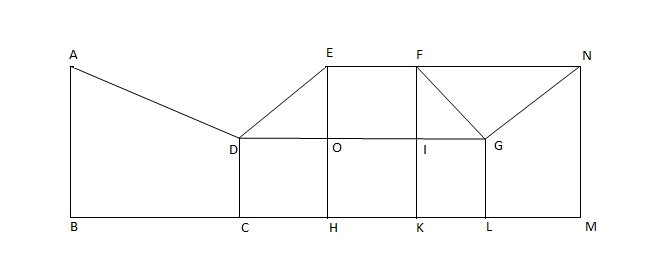### CAT 2002 Question Paper Question 6

Instructions

Directions for the next two questions: Answer the questions based on the following diagram

In the following diagram, $$\angle{ABC}$$ = 90° = $$\angle{DCH}$$ = $$\angle{DOE}$$ = $$\angle{EHK}$$ = $$\angle{FKL}$$ = $$\angle{GLM}$$ = $$\angle{LMN}$$

AB = BC = 2CH = 2CD = EH = FK = 2HK = 4KL = 2LM = MNQuestion 6

# What is the ratio of the areas of the two quadrilaterals ABCD to DEFG?

Solution

Area of ABCD = Area of triangle + Area of rectangle = Let AB = x. So, area = $$3/2 * x^2 / 2 = 3/4 * x^2$$
Area of ODE = $$\frac{1}{2}* \frac{x}{2}*\frac{x}{2}$$=$$x^2/8$$
Area of OEFI = $$\frac{x}{2}*\frac{x}{2}$$=$$x^2/4$$
Area of FGI = $$x^2/16$$
Total area of DEFG = $$7x^2/16$$
Required ratio = 12:7